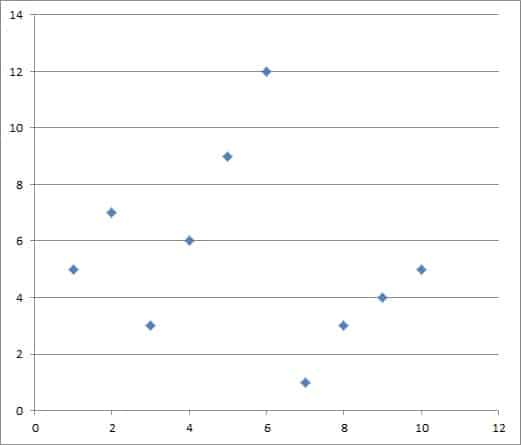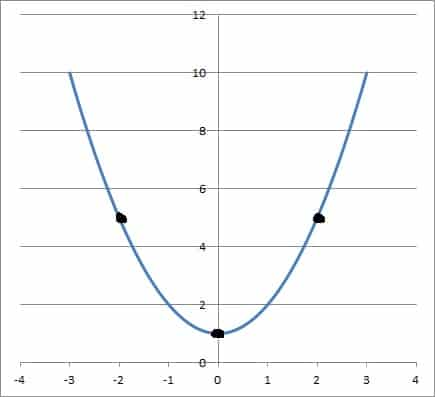# The Difference Between Functions & Equations (3 Key Ideas)

Functions and equations are both used often in mathematics.  However, there is still the question of how these two concepts are related – and whether they are the same thing.

So, what is the difference between functions and equations?  A function has at least 2 variables: an output variable and one or more input variables.  An equation states that two expressions are equal, and it may involve any number of variables (none, one, or more).  A function can often be written as an equation, but not every equation is a function.

Of course, an equation can be very simple (such as 1 + 2 = 3), and it need not contain any variables at all.  Some equations express a relation that is not a function.

In this article, we’ll talk about the difference between functions and equations.  We’ll also look at some examples to help make the ideas clear.

Let’s begin.

Having math trouble?

Looking for a tutor?

## What Is The Difference Between Functions & Equations?

The main difference is that a function always has two or more variables, while an equation may have 0, 1, or more variables.

Many functions can be written as an equation, but not every equation represents a function.  In particular, an equation with less than 2 variables cannot represent a function.

### What Is An Equation?

An equation is a mathematical statement that two expressions are equal.  It always contains the equality symbol (equal sign), or “=”.

An expression is a combination of symbols (numbers, variables, exponents, parentheses, etc.)  We can evaluate an expression   with particular values for each of the variables that appear.

An equation with variables can have zero, one, or more solutions.

#### Examples Of Equations

Equations can range from simple to absurdly complicated.  Here are some examples of equations:

• 1 + 2 = 3  [an equation with no variables]
• x + 4 = 7  [an equation in one variable]
• 2x + 3y = 18  [a linear equation in two variables – this represents a linear function!]
• log10(x) + log10(y2) = 5  [a logarithm equation in two variables]
• 2x3 + 5y4 – 9z2 = 4x + 8y + z – 20  [an equation in three variables]
• x + 3 = x + 4  [an equation in one variable with no solution, since 3 is not equal to 4]

For the last example, we can easily see that there is no solution by subtracting x from both sides.  This gives us 3 = 4, which is always a false statement, no matter the value of the variable x.

Remember that some equations will represent functions (or relations), but not all of them will.

### What Is A Function?

A function is a specific type of relation, where each input has only one output.  A relation is a set of ordered pairs (or triples, or quadruples, or n-tuples for more input variables).

#### Examples Of Functions

Some functions are easy to write as equations, such as:

Some functions are a little more difficult to write as an equation.  For example, a piecewise function has two or more parts that we must write out separately:

The graph of a scatterplot where each input has only one output is also a function.  However, it would be terribly time-consuming to write out a piecewise function for a large number of data points.

It is much faster and easier to present the data as a graph, or perhaps as a table of input and output values – see below.A scatterplot of a function. It is much easier to represent a function this way than with an equation (or set of equations) or a piecewise function.

## How To Tell If An Equation Is A Function

If we can graph a relation from an equation, then we can use the vertical line test to determine whether the graph is a function or not.

The vertical line test states that:

• If any vertical line intersects the graph of a relation more than once, then the relation is not a function.
• Otherwise, the relation is a function (each vertical line intersects the graph of the relation at most once – that is, one time or zero times).

This concept will become clear with some examples of each case.

### Examples Of Relations That Are Not Functions

Here are some examples of relations that are not functions (since they fail the vertical line test).

#### Example 1: x4 = y4

The relation x4 = y4 is not a function.  This is easy to see, even without graphing.

For example, the ordered pairs (1, 1) and (1, -1) are both solutions to the equation x2 = y2 because:

• 14 = 14  [1 = 1]
• 14 = (-1)4  [1 = 1, since a negative number raised to any even power is positive]

We know that (1, 1) and (1, -1) have the same input (x value) but different outputs (v values).  So, they are two different points on the same vertical line.

Thus, this relation fails the vertical line test, and so it is not a function.

#### Example 2: The Unit Circle

The unit circle is represented by the equation x2 + y2 = 1.  However, this relation is not a function.

We can see this without graphing.  For example, the ordered pairs (0, 1) and (0, -1) are both solutions to the equation x2 + y2 = 1 because:

• 02 + 12 = 0 + 1 = 1
• 02 + (-1)2 = 0 + 1 = 1  [since a negative number raised to any even power is positive]

We know that (0, 1) and (0, -1) have the same input (x value) but different outputs (v values).  So, they are two different points on the same vertical line.

Thus, this relation fails the vertical line test, and so it is not a function.

You can easily verify this with the graph of the unit circle, pictured below.The unit circle. The vertical line x = 0 through the origin intersects the units circle twice, meaning it fails the vertical line test (a circle is not a function).

Having math trouble?

Looking for a tutor?

### Examples Of Relations That Are Functions

Here are some examples of relations that are functions (since they pass the vertical line test).

#### Example 1: The Line y = 2x

The line y = 2x has only one output (y) for every input (x).  This is easy to verify if we look at the graph of the line y = 2x, pictured below.

Any vertical line x = a we draw will intersect the line at only one point: (a, 2a).  Thus, the relation passes the vertical line test, and so it is a function (a linear function, to be precise).

#### Example 2: The Parabola y = x2 + 1

The parabola y = x2 + 1 has only one output (y) for every input (x).  This is easy to verify if we look at the graph of the parabola y = x2 + 1, pictured below.

Any vertical line x = a we draw will intersect the parabola at only one point: (a, a2 + 1).  Thus, the relation passes the vertical line test, and so it is a function (a quadratic function, to be precise).

### How To Tell If A Function Is One To One

If we can graph a function from an equation, then we can use the horizontal line test to determine whether the function is one to one (injective) or not.

The horizontal line test states that:

• If any horizontal line intersects the graph of a function more than once, then the function is not one to one.
• Otherwise, the function is a one to one (each horizontal line intersects the graph of the function at most once – that is, one time or zero times).

This concept will become clear with some examples of each case.

### Examples Of Functions That Are Not One To One

Here are some examples of functions that are not one to one (since they fail the horizontal line test).

#### Example 1: The Parabola y = x2 + 1

The parabola y = x2 + 1 is not one to one, since there are two inputs (x values) that lead to the same output (y value).  This is easy to verify if we look at the graph of the parabola y = x2 + 1, pictured below.The graph of the parabola parabola y = x2 + 1, which is not one to one.

The horizontal line y = 1 will intersect the parabola at two points: (1, 2) and (-1, 2).  Thus, the function fails the horizontal line test, and so it is not one to one.

#### Example 2: The Horizontal Line y = 5

The horizontal line y = 5 is not one to one, since there are two inputs (x values) that lead to the same output (y value).  This is easy to verify if we look at the graph of the parabola y = 5, pictured below.

The horizontal line y = 5 will intersect the line y = 5 at infinitely many points.  Thus, the function fails the horizontal line test, and so it is not one to one.

### Examples Of Functions That Are One To One

Here are some examples of functions that are one to one (since they pass the horizontal line test).

#### Example 1: The Line y = 2x

The line y = x passes the horizontal line test.  This is easy to verify if we look at the graph of the line y = 2x, pictured below.

Any horizontal line y = b we draw will intersect the line at only one point: (b, b).  Thus, the function passes the horizontal line test, and so it is one to one.

#### Example 2: The Cubic y = x3

The cubic function y = x3 passes the horizontal line test.  This is easy to verify if we look at the graph of the line y = x3, pictured below.

Any horizontal line y = b we draw will intersect the line at only one point: (b1/3, b).  Since b is a real number, it has only one real cube root (the other two are complex).

Thus, the function passes the horizontal line test, and so it is one to one.

## Conclusion

Now you know the main differences between a function and an equation.  You also have some examples to help you remember what each one looks like.